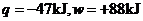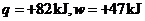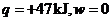Chapter 10, Problem 79AP### Introductory Chemistry: A Foundati...

9th Edition
Steven S. Zumdahl + 1 other
ISBN: 9781337399425

#### Solutions

Chapter
Section### Introductory Chemistry: A Foundati...

9th Edition
Steven S. Zumdahl + 1 other
ISBN: 9781337399425
Textbook Problem
3 views

# Calculatetor each of the following.l type='a'>i>i>In which of these cases do the surroundings do work on the system?

Interpretation Introduction

(a)

Interpretation:

The internal energy change should be calculated.

Concept Introduction:

According to 1st law of thermodynamics the internal energy change results in heat change along with some work.

Explanation

As per 1st law of thermodynamics we can write ΔE=q+w=47

Interpretation Introduction

(b)

Interpretation:

The internal energy change must be calculated.

Concept Introduction:

According to 1st law of thermodynamics the internal energy change results in heat change along with some work.

Interpretation Introduction

(c)

Interpretation:

The internal energy change must be calculated.

Concept Introduction:

According to 1st law of thermodynamics the internal energy change results in heat change along with some work.

Interpretation Introduction

(d)

Interpretation:

In which of the given cases the surroundings do work on the system.

Concept Introduction:

Work done on the system is considered as positive.

### Still sussing out bartleby?

Check out a sample textbook solution.

See a sample solution

#### The Solution to Your Study Problems

Bartleby provides explanations to thousands of textbook problems written by our experts, many with advanced degrees!

Get Started